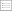Print this page

DISCUSSION PAPER PI-9811

Some Notes on the Dynamics and Optimal Control of Stochastic
Pension Fund Models in Continuous Time

Andrew Cairns

Heriot-Watt University, Edinburgh

ABSTRACT

This paper discusses the modelling and control of pension funds.

A continuous-time stochastic pension fund model is proposed in which there
are n risky assets plus the risk-free asset as well as randomness in the level
of benefit outgo. We consider Markov control strategies which optimise over
the contribution rate and over the range of possible asset-allocation strategies.

For a general (not necessarily quadratic) loss function it is shown that the optimal
proportions of the fund invested in each of the risky assets remain constant
relative to one another. Furthermore, the asset allocation strategy always lies
on the capital market line familiar from modern portfolio theory.

A general quadratic loss function is proposed which provides an explicit solution
for the optimal contribution and asset-allocation strategies. It is noted that these
solutions are not dependent on the level of uncertainty in the level of benefit outgo,
suggesting that small schemes should operate in the same way as large ones.
The optimal asset-allocation strategy, however, is found to be counterintuitive
leading to some discussion of the form of the loss function.

The stationary distribution of the process is considered and optimal strategies
compared with dynamic control strategies.

Finally there is some discussion of the effects of constraints on contribution and
asset-allocation strategies.

ISSN 1367-580x.